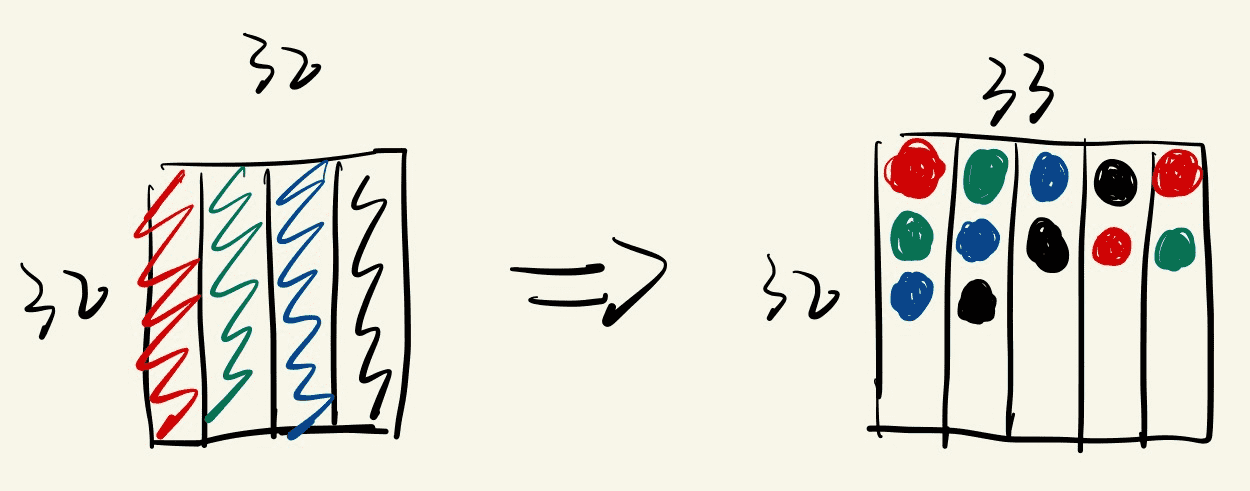# 使用全局内存进行矩阵转置

__global__ void matrix_trans_kernel(int *in, int *out) {
int i = blockIdx.x * blockDim.x + threadIdx.x;
int j = blockIdx.y * blockDim.y + threadIdx.y;
if (i < N && j < N) {
out[i * N + j] = in[j * N + i];  // 顺序读，非顺序写
// out[j * N + i] = in[i * N + j];  // 顺序写，非顺序读
}
}

# 使用共享内存优化矩阵转置

const int BLOCK_SIZE = 32;

__global__ void matrix_trans_kernel_1(int *in, int *out) {
__shared__ int buffer[BLOCK_SIZE][BLOCK_SIZE];

int offset_x = blockIdx.x * blockDim.x;
int offset_y = blockIdx.y * blockDim.y;

int j = offset_x + threadIdx.x;
int i = offset_y + threadIdx.y;
if (i < N && j < N) {
}

if (i < N && j < N) {
}
}

# 优化 bank 冲突# 参考

《CUDA 编程：基础与实践》（樊哲勇，清华大学出版社）